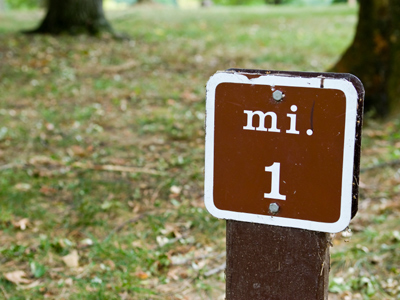What is 1 mile equal to in kilometres?

# Measures (Very Easy)

Nowadays, we use the metric system: metres for length, kilograms for weight and litres for volume. Do you know your metric units?

The following symbols may be of use in doing this quiz:

• millimetre (mm); centimetre (cm); metre (m)
• milligram (mg); gram (g); kilogram (kg)
• square millimetre (mm2); square centimetre (cm2); square metre (m2)
• cubic millimetre (mm3); cubic centimetre (cm3); cubic metre (m3)
• millilitre (ml or mL); litre (l or L)

Do this 11-plus Maths quiz and see if you measure up to the challenge.

1.
What is 1 mile equal to in kilometres?
1.75 km
1 km
2.2 km
1.61 km
1 mile = 1.61 km = 1,610 m (for practical purposes, use 1.6 km). You should know this because miles are still used in many countries of the world
2.
How many kilograms are there in a metric ton?
100,000 (one hundred thousand)
10,000
1,000
100
1,000 kg = 1 t. A 'metric ton' is also written as 'tonne'
3.
How many millimetres are there in a centimetre?
100
10
1,000
10,000
10 mm in 1 cm
4.
How many square metres are there in an acre?
40,000
4,100
4,047
5,000
4,047 square metres = 1 acre (for practical purposes, use 4,000 square metres). This has been included because 'acre' is still used in many parts of the world: farmers use it! By the way, 1 hectare = 10,000 square metres = 2.471 acres (for practical purposes, use 2.5 acres)
5.
How many millilitres are there in one litre?
100,000
10,000
100
1,000
1,000 ml in 1 l. Note: 1 cubic centimetre = 1 ml
6.
How many square metres are there in one square kilometre?
1,000,000 (one million)
10,000
1,000
100,000 (one hundred thousand)
1,000 m in 1 km. One square kilometre = 1,000 × 1,000 = 1,000,000 square metres
7.
How many cubic centimetres are there in a litre?
100
1,000
10,000
10
1,000 cubic cm in 1 litre
8.
How many grams are there in a kilogram?
10
10,000
1,000
100
1,000 g in 1 kg
9.
How many metres are there in a kilometre?
10
100
1,000
10,000
1,000 metres in a kilometre
10.
How many cubic centimetres are there in one cubic metre?
1,000,000 (one million)
10,000
1,000
100,000 (one hundred thousand)
100 cm in 1 m. A cubic metre = 100 × 100 × 100 = 1,000,000 cubic centimetres
Author:  Frank Evans

We use cookies to make your experience of our website better.

To comply with the new e-Privacy directive, we need to ask for your consent -